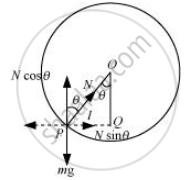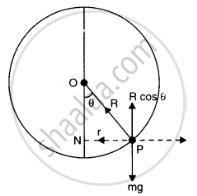# A Thin Circular Loop of Radius R Rotates About Its Vertical Diameter with an Angular Frequency ω What is the Angle Made by the Radius Vector Joining the Centre to the Bead with the Vertical Downward Direction - Physics

#### Question

A thin circular loop of radius rotates about its vertical diameter with an angular frequency ωShow that a small bead on the wire loop remains at its lowermost point for omega <= sqrt(g/R) .What is the angle made by the radius vector joining the centre to the bead with the vertical downward direction for omega = sqrt("2g"/R) ?Neglect friction.

#### Solution 1

Let the radius vector joining the bead with the centre make an angle θ, with the vertical downward direction.OP = R = Radius of the circle

N = Normal reaction

The respective vertical and horizontal equations of forces can be written as:

mg = Ncosθ ... (i)

mlω2 = Nsinθ … (ii)

In ΔOPQ, we have:

sin theta = l/R

l = Rsin theta...(iii)

Substiting  equation (iii) in equation (ii) we get

m(Rsinθω2 = Nsinθ

mR ω2 = N ... (iv)

Substituting equation (iv) in equation (i), we get:

mg = mR ω2 cosθ

cos theta = g/(Romega^2) ...(V)

Since cosθ ≤ 1, the bead will remain at its lowermost point for g/(Romega^2) <= 1 i.e for omega <= sqrt(g/R)

For omega = sqrt((2g)/R) or omega^2 =  ((2g)/R)  ..(vi)

On equating equations (v) and (vi), we get:

(2g)/R =  g/(Rcos theta)

cos theta  = 1/2

:. theta  = cos^(-1) (0.5 ) = 60^@

#### Solution 2

Let the radius vector joining the bead to the centre of the wire make an angle theta with the verticle downward dirction. if N is normal reaction, then from fig.

mg  = N cos theta  ....(i)

mromega^2 = N sin theta   ...(ii)

or m(R sin theta) omega^2 = N sin theta

ormRomega^2 =  N

or cos theta =  g/(Romega^2)

As |cos theta| <= 1, therefore bead will remain at its lowermost point for

g/(Romega^2) <= 1 or omega <= sqrt(g/R)

When omega = sqrt((2g)/R) from equation iii

cos theta = g/R(R/"2g") = 1/2

theta = 60^@Is there an error in this question or solution?